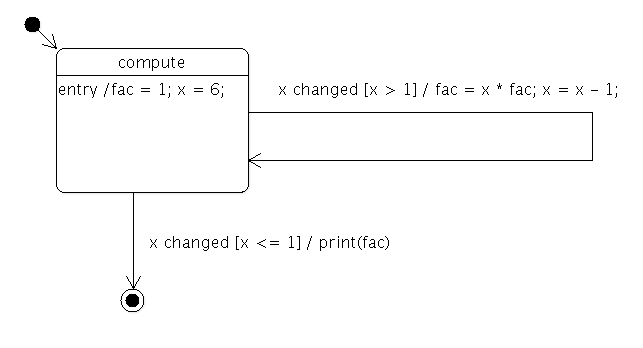·  Page d'accueil  ·  Toutes les classes  ·  Toutes les fonctions  ·  Vues d'ensemble  ·

# Factorial States Example

Files:

The Factorial States example shows how to use The State Machine Framework to calculate the factorial of an integer.

The statechart for calculating the factorial looks as follows:In other words, the state machine calculates the factorial of 6 and prints the result.

``` class Factorial : public QObject
{
Q_OBJECT
Q_PROPERTY(int x READ x WRITE setX)
Q_PROPERTY(int fac READ fac WRITE setFac)
public:
Factorial(QObject *parent = 0)
: QObject(parent), m_x(-1), m_fac(1)
{
}

int x() const
{
return m_x;
}

void setX(int x)
{
if (x == m_x)
return;
m_x = x;
emit xChanged(x);
}

int fac() const
{
return m_fac;
}

void setFac(int fac)
{
m_fac = fac;
}

Q_SIGNALS:
void xChanged(int value);

private:
int m_x;
int m_fac;
};```

The Factorial class is used to hold the data of the computation, x and fac. It also provides a signal that's emitted whenever the value of x changes.

``` class FactorialLoopTransition : public QSignalTransition
{
public:
FactorialLoopTransition(Factorial *fact)
: QSignalTransition(fact, SIGNAL(xChanged(int))), m_fact(fact)
{}

virtual bool eventTest(QEvent *e)
{
if (!QSignalTransition::eventTest(e))
return false;
QStateMachine::SignalEvent *se = static_cast<QStateMachine::SignalEvent*>(e);
return se->arguments().at(0).toInt() > 1;
}

virtual void onTransition(QEvent *e)
{
QStateMachine::SignalEvent *se = static_cast<QStateMachine::SignalEvent*>(e);
int x = se->arguments().at(0).toInt();
int fac = m_fact->property("fac").toInt();
m_fact->setProperty("fac",  x * fac);
m_fact->setProperty("x",  x - 1);
}

private:
Factorial *m_fact;
};```

The FactorialLoopTransition class implements the guard (x > 1) and calculations (fac = x * fac; x = x - 1) of the factorial loop.

``` class FactorialDoneTransition : public QSignalTransition
{
public:
FactorialDoneTransition(Factorial *fact)
: QSignalTransition(fact, SIGNAL(xChanged(int))), m_fact(fact)
{}

virtual bool eventTest(QEvent *e)
{
if (!QSignalTransition::eventTest(e))
return false;
QStateMachine::SignalEvent *se = static_cast<QStateMachine::SignalEvent*>(e);
return se->arguments().at(0).toInt() <= 1;
}

virtual void onTransition(QEvent *)
{
fprintf(stdout, "%d\n", m_fact->property("fac").toInt());
}

private:
Factorial *m_fact;
};```

The FactorialDoneTransition class implements the guard (x <= 1) that terminates the factorial computation. It also prints the final result to standard output.

``` int main(int argc, char **argv)
{
QCoreApplication app(argc, argv);
Factorial factorial;
QStateMachine machine;```

The application's main() function first creates the application object, a Factorial object and a state machine.

```     QState *compute = new QState(&machine);
compute->assignProperty(&factorial, "fac", 1);
compute->assignProperty(&factorial, "x", 6);

The compute state is created, and the initial values of x and fac are defined. A FactorialLoopTransition object is created and added to the state.

```     QFinalState *done = new QFinalState(&machine);
FactorialDoneTransition *doneTransition = new FactorialDoneTransition(&factorial);
doneTransition->setTargetState(done);

A final state, done, is created, and a FactorialDoneTransition object is created with done as its target state. The transition is then added to the compute state.

```     machine.setInitialState(compute);
QObject::connect(&machine, SIGNAL(finished()), &app, SLOT(quit()));
machine.start();

return app.exec();
}```

The machine's initial state is set to be the compute state. We connect the QStateMachine::finished() signal to the QCoreApplication::quit() slot, so the application will quit when the state machine's work is done. Finally, the state machine is started, and the application's event loop is entered.Publicitй

## Best Of

Semaine
Mois
Annйe

### Le Qt Developer Network au hasard#### Combiner licence, а propos et fermer d'une derniиre maniиre

Le Qt Developer Network est un rйseau de dйveloppeurs Qt anglophone, oщ ils peuvent partager leur expйrience sur le framework. Lire l'article.

### Contact

• Vous souhaitez rejoindre la rйdaction ou proposer un tutoriel, une traduction, une question... ? Postez dans le forum Contribuez ou contactez-nous par MP ou par email (voir en bas de page).

### Qt dans le magazine

 Cette page est une traduction d'une page de la documentation de Qt, йcrite par Nokia Corporation and/or its subsidiary(-ies). Les йventuels problиmes rйsultant d'une mauvaise traduction ne sont pas imputables а Nokia. Qt 4.6 Copyright © 2012 Developpez LLC. Tous droits rйservйs Developpez LLC. Aucune reproduction, mкme partielle, ne peut кtre faite de ce site et de l'ensemble de son contenu : textes, documents et images sans l'autorisation expresse de Developpez LLC. Sinon, vous encourez selon la loi jusqu'а 3 ans de prison et jusqu'а 300 000 E de dommages et intйrкts. Cette page est dйposйe а la SACD. Vous avez dйnichй une erreur ? Un bug ? Une redirection cassйe ? Ou tout autre problиme, quel qu'il soit ? Ou bien vous dйsirez participer а ce projet de traduction ? N'hйsitez pas а nous contacter ou par MP !

Partenaires

Hйbergement Web